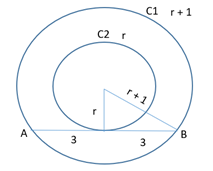# Let C1 and C2 be concentric circles such that - Quantitative Aptitude - CAT Mensuration

## CAT 2020 - Slot 2 - Quantitative Aptitude - Mensuration - Question 21 - Let C1 and C2 be concentric circles such that

Q.21: Let C1 and C2 be concentric circles such that the diameter of C1 is 2cm longer than that of C2. If a chord of C1 has length 6 cm and is a tangent to C2, then the diameter, in cm of C1 isThe line from the center bisects chohrd of length 6

into 3 and 3. Since the triangle is of 3, r and r +1

Triplet of triangle 3,4 and 5

r = 4, r + 1 = 5 (radius of C1)

Diameter of C1 = 10

### Past Year Question Paper & SolutionsCounselling Session
By IIM Mentor

#### Free Material Area